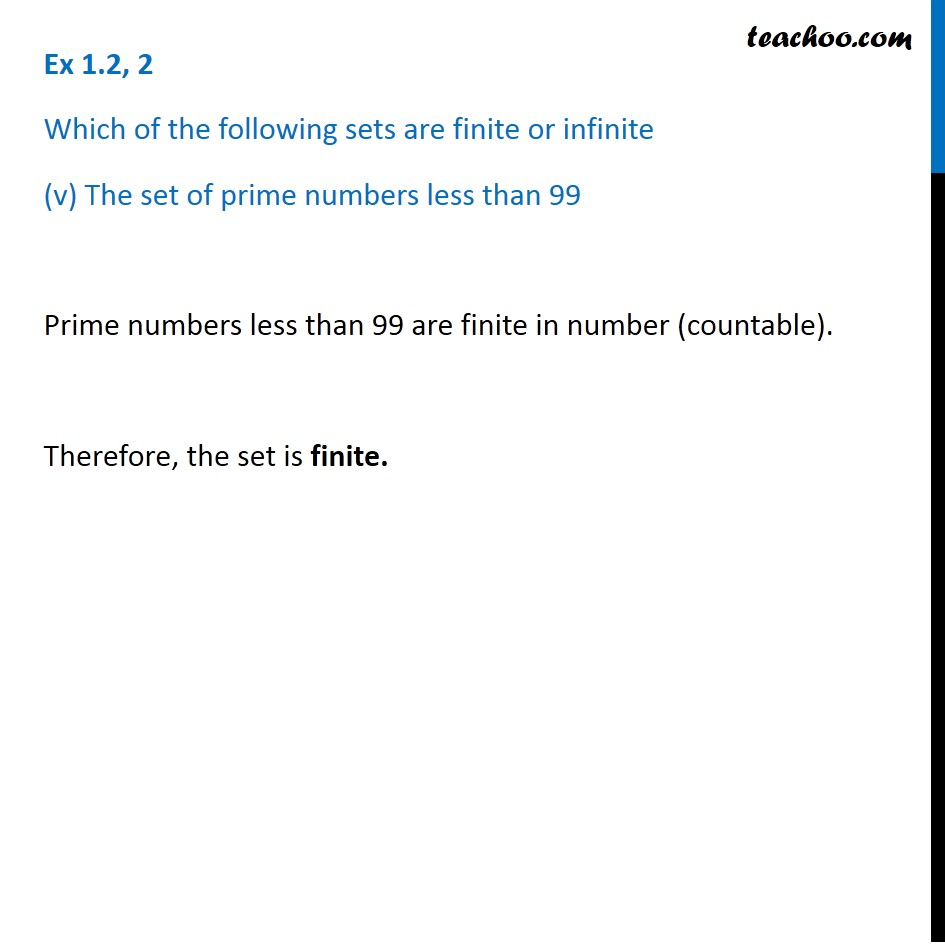1. Chapter 1 Class 11 Sets (Term 1)
2. Serial order wise
3. Ex 1.2

Transcript

Ex 1.2, 2 Which of the following sets are finite or infinite (v) The set of prime numbers less than 99 Prime numbers less than 99 are finite in number (countable). Therefore, the set is finite.